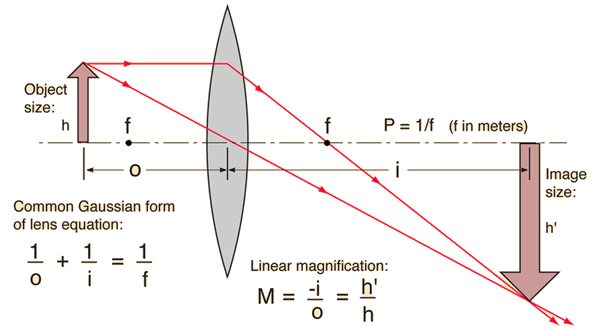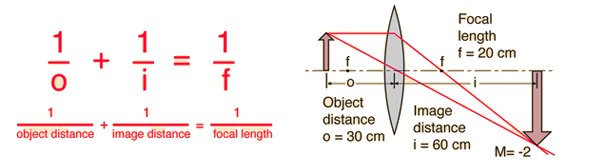Real Image Formation

If a luminous object is placed at a distance greater than the focal length away from a convex lens, then it will form an inverted real image on the opposite side of the lens. The image position may be found from the lens equation or by using a ray diagram provided that it can be considered a "thin lens".Enter data below, then click on the quantity you wish to calculate in the active formula above.
For a lens of focal length f = cm,
corresponding to lens power P = diopters,
an object distance of o = cm
will produce an image at i = cm.
The linear magnification will be M = .

If the lens equation yields a negative image distance, then the image is a virtual image on the same side of the lens as the object. If it yields a negative focal length, then the lens is a diverging lens rather than the converging lens in the illustration. The lens equation can be used to calculate the image distance for either real or virtual images and for either positive on negative lenses. The linear magnification relationship allows you to predict the size of the image.

Index

Lens concepts

 HyperPhysics***** Light and Vision R Nave
Go Back